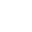### question exercise 6 5 part level submission date explanation unit cost calculate the 4279946Question

Exercise 6-5 (Part Level Submission)

Date

Explanation

Unit Cost

Calculate the weighted-average unit cost. (Round answer to 3 decimal places, e.g. 5.125.)

Calculate the ending inventory at May 31 using the FIFO, LIFO and average-cost methods. (Round answers to 0 decimal places, e.g. 125.)

FIFO

LIFO

AVERAGE-COST

Exercise 6-5 (Part Level Submission)

Carla Vista Co. uses a periodic inventory system. Its records show the following for the month of May, in which 70 units were sold.

 Date Explanation Units Unit Cost Total Cost May 1 Inventory 32 \$8 \$256 15 Purchase 24 9 216 24 Purchase 39 10 390 Total 95 \$862